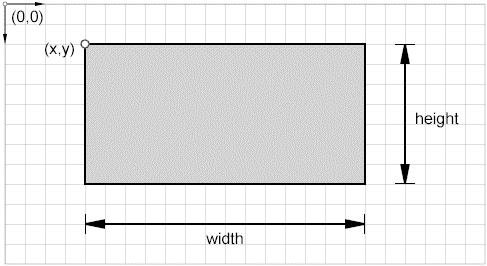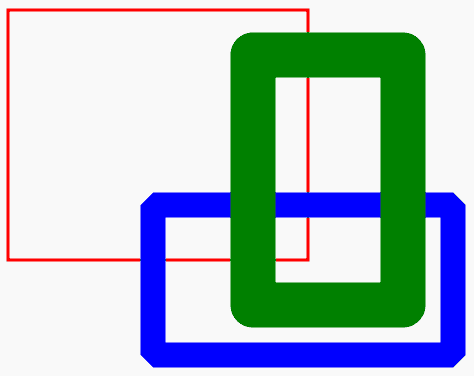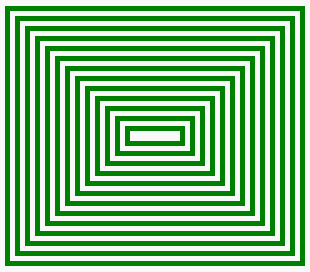# HTML5 Canvas Rectangle

## Draw Rectangles

To draw a rectangle, specify the x and y coordinates (upper-left corner) and the height and width of the rectangle. There are three rectangle methods :

• fillRect()
• strokeRect()
• clearRect()## fillRect() Method

The fillRect() method is used to fill a rectangle in the current color, gradient, or pattern.

Syntax :

`ctx.fillRect(x, y, width, height)`
Parameters Type Description

x

number The x-coordinate (in pixels), the upper-left corner of the rectangle in relation to the coordinates of the canvas.
y number The y-coordinate (in pixels), of the upper-left corner of the rectangle in relation to the coordinates of the canvas.
width number The width (in pixels), of the rectangle.
height number The height (in pixels), of the rectangle.

Remark : If the width or height parameter is zero, this method has no effect.

Example : HTML5 Canvas rectangle using fillRect() Method

The following web document fills a rectangle by fillStyle property.

Output:Code:

``````<!DOCTYPE html>
<html>
<title>Rectangle example</title>
<body>
<canvas id="DemoCanvas" width="500" height="600"></canvas>
<script>
var canvas = document.getElementById("DemoCanvas");
if (canvas.getContext)
{
var ctx = canvas.getContext('2d');
ctx.fillStyle='#fa4b2a';    // color of fill
ctx.fillRect(10, 40, 140, 160); // create rectangle
}
</script>
</body>
</html>``````

## strokeRect() Method

The strokeRect() method is used to fill a rectangle in the current color, gradient, or pattern.

Syntax :

`ctx.strokeRect(x, y, width, height)`
Parameters Type Description
x number The x-coordinate (in pixels), the upper-left corner of the rectangle in relation to the coordinates of the canvas.
y number The y-coordinate (in pixels), of the upper-left corner of the rectangle in relation to the coordinates of the canvas.
width number The width (in pixels), of the rectangle.
height number The height (in pixels), of the rectangle.

Example: HTML5 Canvas rectangle using fillRect() Method

The web document draws rectangles by using strokeStyle, lineJoin, and lineWidth for various effects.

Output:Code:

``````<!DOCTYPE html>
<html>
<title>Rectangle example</title>
<body>
<canvas id="DemoCanvas" width="500" height="600"></canvas>
<script>
var canvas = document.getElementById("DemoCanvas");
if (canvas.getContext)
{
var ctx = canvas.getContext('2d');
ctx.lineWidth = "3";
ctx.strokeStyle = "red";
ctx.strokeRect(5, 5, 300, 250);
ctx.lineWidth = "25";
ctx.lineJoin = "bevel";
ctx.strokeStyle = "blue";
ctx.strokeRect(150, 200, 300, 150);
ctx.lineJoin = "round";
ctx.lineWidth = "45";
ctx.strokeStyle = "green";
ctx.strokeRect(250, 50, 150, 250);
}
</script>
</body>
</html>``````

## clearRect() Method

The clearRect() method is used to clear a rectangle (erase the area to transparent black).

Syntax :

`ctx.clearRect(x, y, width, height)`
Parameters Type Description

x

number The x-coordinate (in pixels), the upper-left corner of the rectangle in relation to the coordinates of the canvas.
y number The y-coordinate (in pixels), of the upper-left corner of the rectangle in relation to the coordinates of the canvas.
width number The width (in pixels), of the rectangle.
height number The height (in pixels), of the rectangle.

Example: HTML5 Canvas rectangle using clearRect() Method

The web document draws a series of rectangles using fillRect() and clearRect() methods.

Output:Code:

``````<!DOCTYPE html>
<html>
<title>Rectangle example</title>
<body>
<canvas id="DemoCanvas" width="500" height="600"></canvas>
<script>
var canvas = document.getElementById("DemoCanvas");
if (canvas.getContext)
{
var ctx = canvas.getContext('2d');
ctx.fillStyle='green';    // color of fill
var y=40, h=300, w=260;
for (var x = 10; x!=140; x=x+10)
{
ctx.fillRect(x, y, h, w);
ctx.clearRect(x+5, y+5, h-10, w-10);
y=y+10;
h=h-20;
w=w-20;
}
}
</script>
</body>
</html>``````

Code Editor:

See the Pen html css common editor by w3resource (@w3resource) on CodePen.

﻿